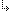- 2.2.9.1. -2. TMT Pascal Language Description2.2. Pascal Language Structure2.2.9. Statements2.2.9.1. Assigments

## 2.2.9.1. Assigments

An assignment assigns a value to a variable. An assignment takes the following form:
`variable := expression;`
where the value returned by expression is stored in variable.

The type of the value returned by expression must be compatible with the type of variable. If variable appears in expression, its value is the value prior to the assignment. The following are examples of assignments:
```const
Letter    = 'A';
var
Alpha     : Char;
Value, i  : Integer;
l         : Longint;
s         : String;
begin
Alpha     := Letter;
s         := 'A string variable';
Value     := \$643F;
i         := 2675;
l         := 200 + (Value * i);
end.```

- 2.2.9.1. -